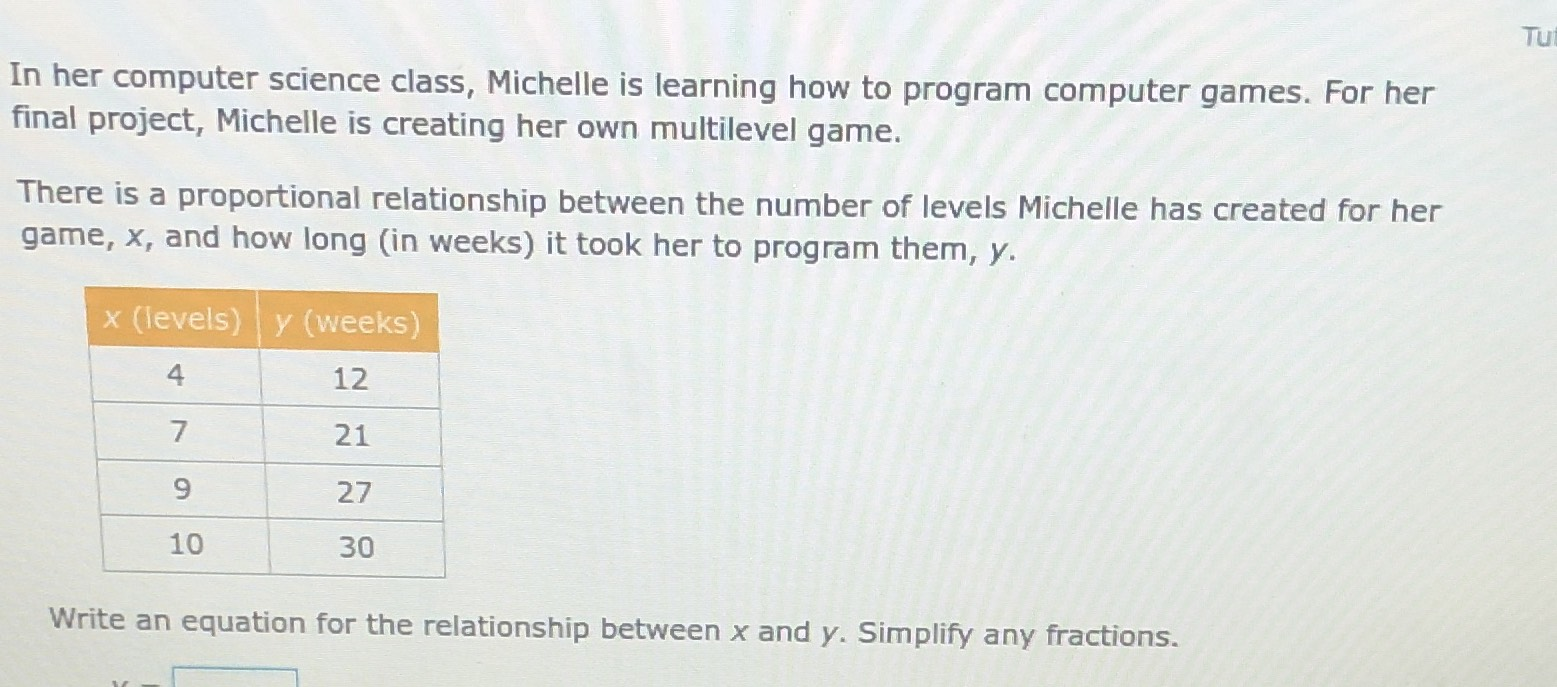### Still have math questions?

Algebra
QuestionIn her computer science class, Michelle is learning how to program computer games. For her final project, Michelle is creating her own multilevel game. There is a proportional relationship between the number of levels Michelle has created for her game, $$x$$ , and how long (in weeks) it took her to program them, $$y$$ .

Write an equation for the relationship between $$x$$ and $$y$$ . Simplify any fractions.